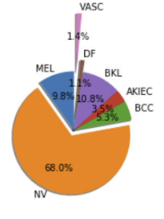# Weights in weighted loss (nn.CrossEntropyLoss)

Hello Altruists,
I am working on a multiclass classification with image data. The training set has 9015 images of 7 different classes.
Target labeling looks like `0,1,0,0,0,0,0`
But the dataset is very much skewed to one class having 68% images and lowest amount is 1.1% belongs to another class. Please take a look at the figure below:How can I use weighted `nn.CrossEntropyLoss` ?
Do I normalize the weights in order as it is or in reverse order?

``````weights = [9.8, 68.0, 5.3, 3.5, 10.8, 1.1, 1.4] #as class distribution
class_weights = torch.FloatTensor(weights).cuda()
Criterion = nn.CrossEntropyLoss(weight=class_weights)
``````
2 Likes

Hello,

I do not know what you mean by reverser order, but I think it is better if you normalize the weights proportionnally to the reverse of the initial weights (so the more examples you have in the training data, the smaller the weight you have in the loss). Here is what I would do:

``````    weights = torch.tensor([9.8, 68.0, 5.3, 3.5, 10.8, 1.1, 1.4], dtype=torch.float32)
weights = weights / weights.sum()
print(weights)
weights = 1.0 / weights
weights = weights / weights.sum()
print(weights)
``````
3 Likes

Hey thanks!
I did the following weighing which gave me pretty good results:

``````nSamples = [887, 6130, 480, 317, 972, 101, 128]
normedWeights = [1 - (x / sum(nSamples)) for x in nSamples]
normedWeights = torch.FloatTensor(normedWeights).to(device)
``````

Yes you are right

the more instance the less weight of a class

I will also try the way you’ve mentioned.

And are there ways to optimize weights?
Thanks again!

4 Likes

Yes, it seems to be possible. I found this thread which explains how you can learn the weights for the cross-entropy loss: Is that possible to train the weights in CrossEntropyLoss?

Good luck!

1 Like